Dr. J's Maths.com
Where the techniques of Maths
are explained in simple terms.

Trigonometry - Measuring angles - finding the size of an angle.
Test Yourself 1.

 Find the size of the angle indicated for each question (correct to the nearest degree). 1.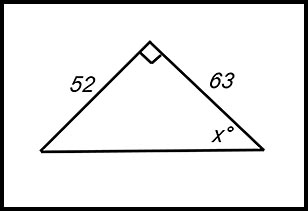Answer.40o. 2.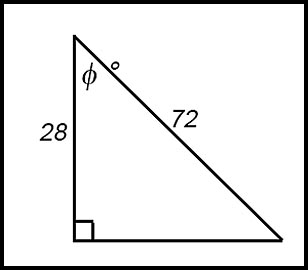Answer.67o. 3.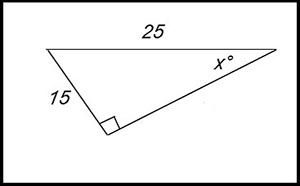Answer.37o. 4.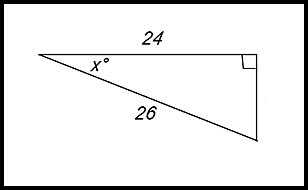5.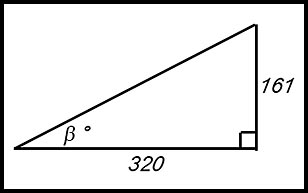6.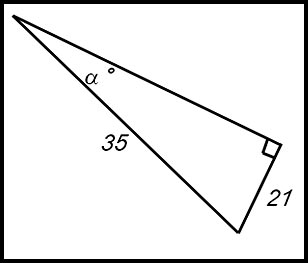Find the size of the angle indicated for each question (correct to the nearest minute). 7.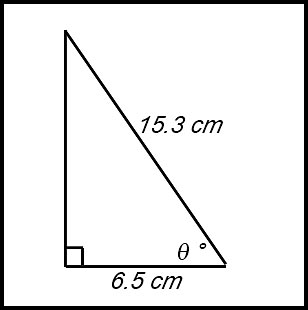Answer.64o 52'. 8.Answer.59o 7'. 9.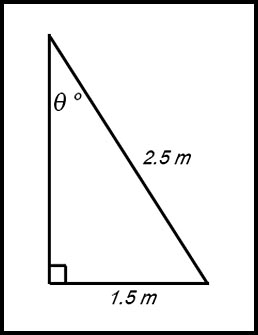Answer.36o 52'. 10.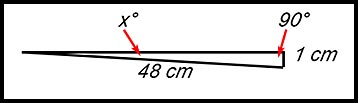Answer.1o 12'. Problems. 15. I have two pictures on one of my walls.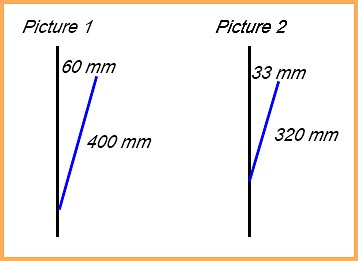The diagram shows the distances below the anchoring point to hold the pictures and the horizontal distance of the picture from the anchoring point. By calculating the angle each picture makes to the wall, comment on the inclination of the picture as seen from the front. 16. The minimum measurements in the Australian Standard for the size of a step are 130 mm wide × 215 mm high. What angle is formed by such a step? 17. My driveway increases in height by 35 cm as I drive 3.4 m.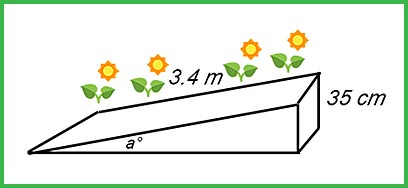At what height is the driveway inclined to the horizontal?